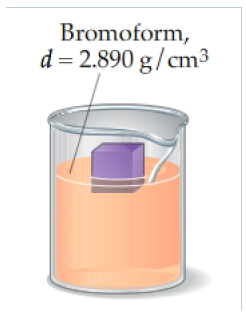# Problem: Based on the sketch, you measure that 40% of the block is submerged. Use the first and third sketches to find the density of the block. Express your answer with the appropriate units.The accompanying sketches suggest four observations made on a small block of plastic material.When analyzing Figure 4, it is helpful to keep in mind that the apparent mass of an object in fluid is equal to its mass in air, minus the mass of the fluid displaced mapparent = mair − mdisplaced fluid

###### FREE Expert Solution
95% (240 ratings)View Complete Written Solution
###### Problem Details

Based on the sketch, you measure that 40% of the block is submerged. Use the first and third sketches to find the density of the block.Express your answer with the appropriate units.

The accompanying sketches suggest four observations made on a small block of plastic material.

When analyzing Figure 4, it is helpful to keep in mind that the apparent mass of an object in fluid is equal to its mass in air, minus the mass of the fluid displaced

mapparent = mair − mdisplaced fluid

Frequently Asked Questions

What scientific concept do you need to know in order to solve this problem?

Our tutors have indicated that to solve this problem you will need to apply the Density of Non-Geometric Objects concept. You can view video lessons to learn Density of Non-Geometric Objects. Or if you need more Density of Non-Geometric Objects practice, you can also practice Density of Non-Geometric Objects practice problems.

What professor is this problem relevant for?

Based on our data, we think this problem is relevant for Professor Tang's class at USF.